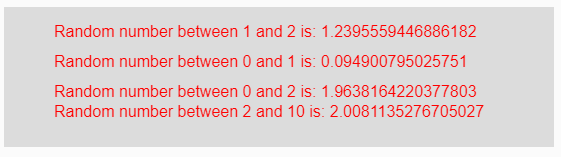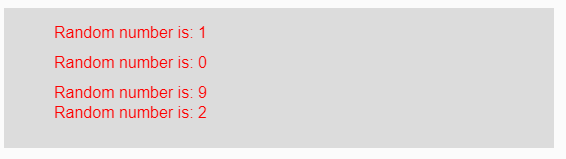# p5.js | randomSeed() Function

The randomSeed() function in p5.js is used to return a random number each time when run the program. The difference between the random() and randomSeed() function is that random() function produces distinct values each time the program is run but when randomSeed() function is used then it gives a constant random number each time the program is run.

Syntax:

`randomSeed( Seed )`

Parameters: This function accepts single parameter Seed which is any integer value.

Return Value: It returns a constant random number.

Below programs illustrate the randomSeed() function in p5.js:

Example 1: This example uses randomSeed() function to return a random number each time when run the program.

 `function` `setup() {  ` `  `  `    ``// Creating Canvas size ` `    ``createCanvas(550, 140);  ` `      `  `    ``// Set the background color  ` `    ``background(220);  ` `   `  `    ``// Calling to randomSeed() function ` `    ``randomSeed(9) ` `     `  `    ``// Calling to random() function with ` `    ``// min and max parameters ` `    ``let A = random(1, 2); ` `    ``let B = random(0, 1); ` `    ``let C = random(2); ` `    ``let D = random(2, 10); ` `     `  `    ``// Set the size of text  ` `    ``textSize(16);  ` `      `  `    ``// Set the text color  ` `    ``fill(color(``'red'``));  ` `    `  `    ``// Getting random number ` `    ``text(``"Random number between 1 and 2 is: "` `+ A, 50, 30); ` `    ``text(``"Random number between 0 and 1 is: "` `+ B, 50, 60); ` `    ``text(``"Random number between 0 and 2 is: "` `+ C, 50, 90); ` `    ``text(``"Random number between 2 and 10 is: "` `+ D, 50, 110); ` `}  `

Output:Note: In the above example, in variable “C” only one parameter is passed then it returns a random number from lower bound 0 to upper bound of that number.

Example 2: This example uses randomSeed() function to return a random number each time when run the program.

 `function` `setup() {  ` `  `  `    ``// Creating Canvas size ` `    ``createCanvas(550, 140);  ` `      `  `    ``// Set the background color  ` `    ``background(220);  ` `    `  `    ``// Calling to randomSeed() function ` `    ``randomSeed(9) ` `     `  `    ``// Calling to random() function with ` `    ``// parameter array of some elements ` `    ``let A = random([1, 2, 3, 4]); ` `    ``let B = random([0, 1]); ` `    ``let C = random([2, 6, 7, 9]); ` `    ``let D = random([2, 10]); ` `     `  `    ``// Set the size of text  ` `    ``textSize(16);  ` `      `  `    ``// Set the text color  ` `    ``fill(color(``'red'``));  ` `    `  `    ``// Getting random number ` `    ``text(``"Random number is: "` `+ A, 50, 30); ` `    ``text(``"Random number is: "` `+ B, 50, 60); ` `    ``text(``"Random number is: "` `+ C, 50, 90); ` `    ``text(``"Random number is: "` `+ D, 50, 110); ` `}     `

Output:My Personal Notes arrow_drop_upCheck out this Author's contributed articles.

If you like GeeksforGeeks and would like to contribute, you can also write an article using contribute.geeksforgeeks.org or mail your article to contribute@geeksforgeeks.org. See your article appearing on the GeeksforGeeks main page and help other Geeks.

Please Improve this article if you find anything incorrect by clicking on the "Improve Article" button below.

Article Tags :

Be the First to upvote.

Please write to us at contribute@geeksforgeeks.org to report any issue with the above content.